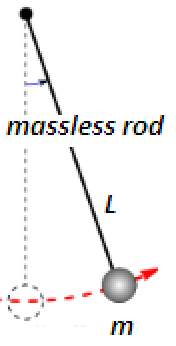# Change in time period of a simple pendulum due to change in temperature Calculator

This Calctown Calculator calculates the change in time period of a simple pendulum due to change in temperature.

K-1
K
s

#### Result

sClick here to view image

where

T-T0 = time lost in T0 time

α = coefficient of linear thermal expansion of rod

Δθ = change in temperature

T0 = initial time period

T = final time period